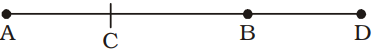## Speed, Time and Distance

#### Speed, Time and Distance

1. A man covers 20 kms in 2 hours.
 Find the distance covered by him in 5 1 hours. 2
1. 50 km
2. 65 km
3. 55 km
4. 45 km

1. Distance = 20 kms
Time = 2 hours

 ∴  Speed = Distance Time

 = 20 = 10 km per hr. 2

Now, we have, Speed = 10 km per hr.
 Time = 11 hr. 2

∴  Distance = Speed × Time
 = 10 × 11 = 55 km 2

##### Correct Option: C

Distance = 20 kms
Time = 2 hours

 ∴  Speed = Distance Time

 = 20 = 10 km per hr. 2

Now, we have, Speed = 10 km per hr.
 Time = 11 hr. 2

∴  Distance = Speed × Time
 = 10 × 11 = 55 km 2

1. Express speed of 60 metres per sec. in km per hour.
1. 232 kmph
2. 216 kmph
3. 116 kmph
4. 118 kmph

1. 60 metres per sec.

 =60 × 18km per hr. 5

= 216 km per hr.

##### Correct Option: B

60 metres per sec.

 =60 × 18km per hr. 5

= 216 km per hr.

1. Express speed of 36 km per hr. in metres per second.
1. 10 m/sec.
2. 8 m/sec.
3. 12 m/sec.
4. 18m/sec.

1. 36 km/hr.

 =36 × 5m/sec. 18

= 10 m/sec.

##### Correct Option: A

36 km/hr.

 =36 × 5m/sec. 18

= 10 m/sec.

1. A man walking at 3 km/hour crosses a square field diagonally in 2 minutes. The area of the field (in square metre) is
1. 3000
2. 5000
3. 6000
4. 2500

1. Speed of person = 3 kmph

 =3000m./min. 60

= 50 m./min.
∴  Length of the diagonal of square field
= 50 × 2 = 100 metre
 ∴  Required time = 1 × (100)2 2

= 5000 sq. metre

##### Correct Option: B

Speed of person = 3 kmph

 =3000m./min. 60

= 50 m./min.
∴  Length of the diagonal of square field
= 50 × 2 = 100 metre
 ∴  Required time = 1 × (100)2 2

= 5000 sq. metre

1. A and B are 15 kms apart and when travelling towards each other meet after half an hour whereas they meet two and a half hours later if they travel in the same direction. The faster of the two travels at the speed of
1. 15 km./hr.
2. 18 km./hr.
3. 10 km./hr.
4. 8 km./hr.

1.A’s speed = x kmph.
B’s speed = y kmph.
When A and B move in opposite
directions they meet at C and
when they move in the same direction, they meet at D.
Case I,
AC + CB = AB

 x + y = 15 2 2

⇒  x + y = 30   ..... (i)
Case II,
 ⇒ x × 5 − y × 5 = 15 2 2

 5 (x – y) = 15 2

 ⇒  x – y = 15 × 2 = 6   .....(ii) 5

∴  On adding equations (i) and (ii),
x + y + x – y = 30 + 6
⇒  2x = 36
 ⇒  x = 36 = 18 kmph. 2

##### Correct Option: BA’s speed = x kmph.
B’s speed = y kmph.
When A and B move in opposite
directions they meet at C and
when they move in the same direction, they meet at D.
Case I,
AC + CB = AB

 x + y = 15 2 2

⇒  x + y = 30   ..... (i)
Case II,